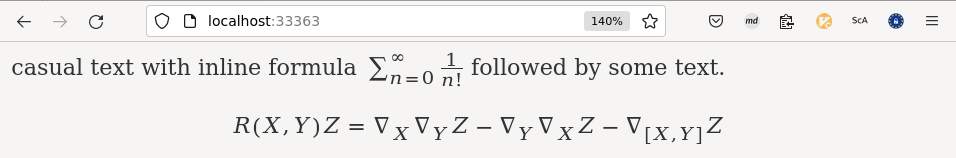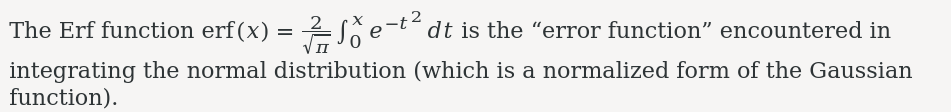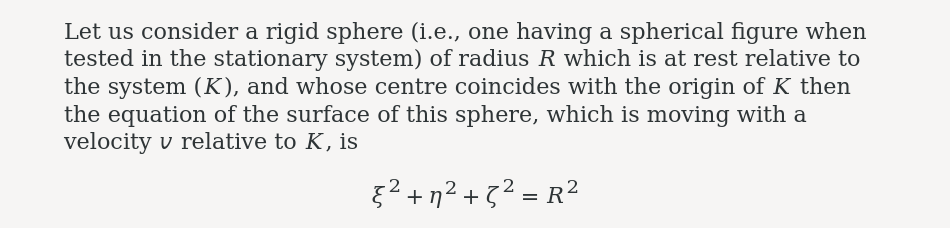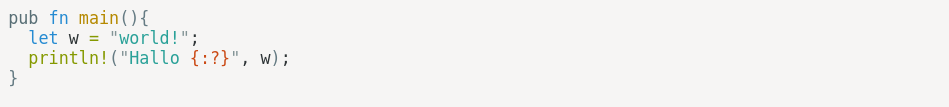One downside when you take notes with your keyboard and laptop is, that you can not enter mathematical formulas.

To remedy this, Tp-Note version 1.19.8 comes with a new feature: its note viewer and internal web-server can now render MathML in note files written in Markdown. It also features syntax highlighting for source code.For this new feature, Tp-Note includes the library Syntect for syntax highlighting and latex2mathml crate that performs the MathML rendition. The following example is taken from latex2mathml's README.md.

## Tp-Note and MathML

Tp-Note's file viewer launches the system's web browser in order to display the rendered content. For the best user experience, it recommended to use Firefox or Safari because both come with full support for MathML enabled by default. The section Choose your favourite web browser as note viewer in Tp-Note's documentation explains how to change the web browser when viewing files. More information about MathML browser compatibility can be found in MathML | MDN.

### Example: Markdown content with inline MathML

Consider the following Markdown input:

The [Erf function]
$\erf ( x ) = \frac{ 2 }{ \sqrt{ \pi } } \int_0^x e^{- t^2} \, dt$
is the "error function" encountered in integrating the [normal distribution]
(which is a normalized form of the [Gaussian function]).


Note, inline equations are always enclosed by $and$. In Tp-Notes built-in viewer the above is rendered into:### Example: Markdown content with a LaTeX equation

The next example adds a LaTeX equation:

> Let us consider a rigid sphere (i.e., one having a spherical
figure when tested in the stationary system) of radius $R$
which is at rest relative to the system ($K$), and whose centre
coincides with the origin of $K$ then the equation of the
surface of this sphere, which is moving with a velocity $v$
relative to $K$, is
math
\xi^2 + \eta^2 + \zeta^2 = R^2



Here the rendered example as it is displayed in Tp-Notes viewer:## Source code syntax highlighting

Another feature of Tp-Note v1.19.8 is the improved syntax highlighting provided by the Syntect crate which supports wide range of programming languages.

The following Markdown excerpt with embedded Rust source code with a “hello world” variant showcases an example:

rust
pub fn main(){
let w = "world!";
println!("Hallo {:?}", w);
}



This is how Tp-Note's viewer renders the embedded source code:Edit 2022-12-16: Update the article and screenshots for Tp-Note version v1.19.8.